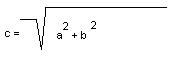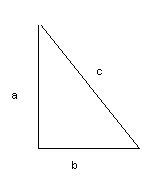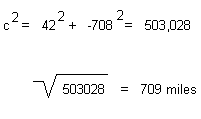# The Pythagorean Theorem

The Pythagorean Theorem computes the hypotenuse of a right triangle using the following relationship between the legs and the hypotenuse of a triangle:

The hypotenuse [c] equals the square root of the sum of the squares of each leg of the triangle [a and b].Example:This is the computed distance in miles.
Use the Pythagorean Theorem with our Picadad values to compute the distance in miles between two zip codes.

Return Back to Calculate Distances